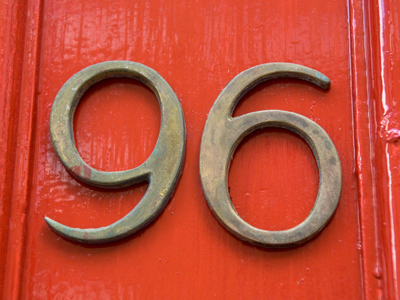12 x 8 = 96.

# 8 Times Table

This Math quiz is called '8 Times Table' and it has been written by teachers to help you if you are studying the subject at elementary school. Playing educational quizzes is an enjoyable way to learn if you are in the 3rd, 4th or 5th grade - aged 8 to 11.

It costs only \$12.50 per month to play this quiz and over 3,500 others that help you with your school work. You can subscribe on the page at Join Us

Learning the 8 times table is all about multiplying numbers by 8. The larger the numbers, the harder it becomes. Learning the 8 times table until you know the answer before you even think about it will really help you when going shopping and multiplying numbers in everyday life.

Play this quiz to test your knowledge of the 8 times table.

1.
7 x 8 =
56
58
62
64
2.
4 x 8 =
18
28
32
37
A fully grown adult has 32 teeth - so long as they haven't lost any!
3.
2 x 8 =
10
12
16
20
4.
9 x 8 =
70
72
74
78
To multiply a number by 9 first times it by 10 and then take it away, so 8 x 10 = 80 and 80 - 8 = 72
5.
11 x 8 =
18
19
80
88
10 x 8 = 80 and 80 + 8 = 88
6.
5 x 8 =
13
18
20
40
To times a number by 5, first times it by 10 and then half it, so 8 x 10 = 80 and 80 ÷ 2 = 40
7.
12 x 8 =
96
108
116
128
8.
6 x 8 =
44
48
52
60
9.
3 x 8 =
22
24
27
29
There are 24 hours in a day - that's 3 lots of 8 hours
10.
8 x 8 =
56
60
64
66
Author:  Amanda Swift